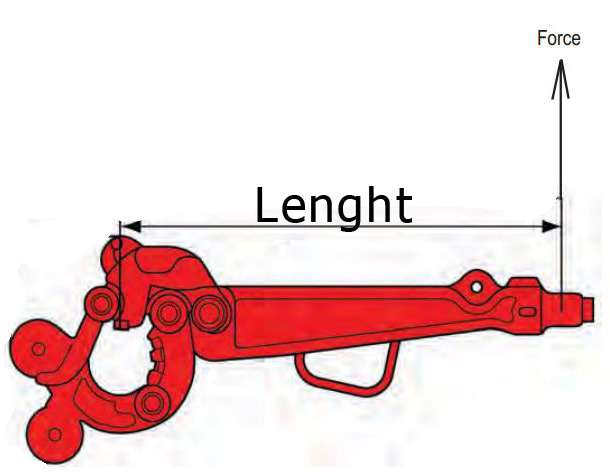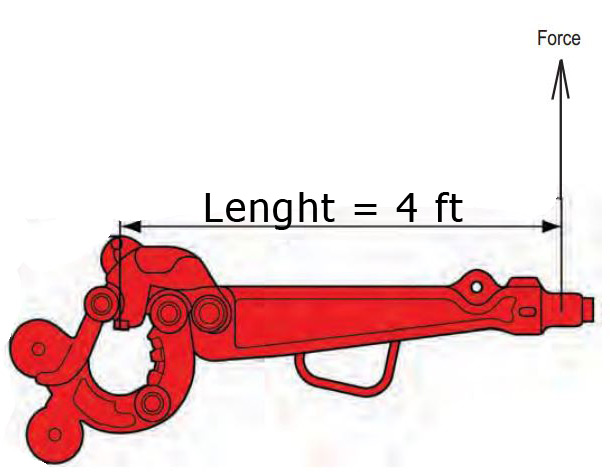## How Much Force Applied to a Rig Tong To Get The Right Torque at The ConnectionOne application of the rig tong is to use to make up connection. The question is asked about how to get the right torque value to the connection if you use the rig tong because you will not see the torque value on the gauge. This article will describe how to determine the correct force applied to get the correct torque value when you use the rig tong to make up the connection.This formula below is used to determine force at required make up torque value.# Oilfield Unit

## Force = Torque ÷ Length of the tong

Where;

Torque in ft-lb

Force in lb

Length of the tong in ft

Force is perpendicular to the tong length.

The illustration above demonstrates the direction of force.

# Metric Unit

## Force = Torque ÷ Length of the tong

Where;

Torque in N-m

Force in

Length of the tong in m

Force is perpendicular to the tong length.

Example:

Connection 4-1/2” IF – required make up torque = 30,700 ft-lb.

Tong length is 4 ftHow much pulling force do you require to achieve the required make up torque?

Force = Torque ÷ Length of the tong

Force = 30,700 ÷ 4.0

Force = 7,675 lb

Answer: 7,675 lb pulling force is applied to 4-ft tong in order to get the torque at connection at 30,700 ft-lb.